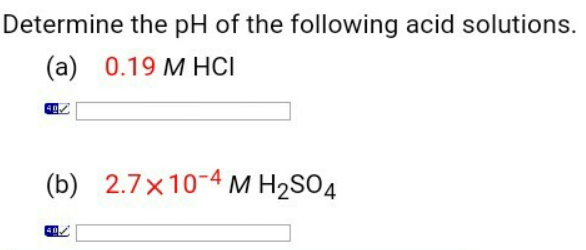Chemistry Practice Problems Strong Acid-Base Calculations Practice Problems Solution: Determine the pH of the following acid solutions.

🤓 Based on our data, we think this question is relevant for Professor Cummings & Dillon & McCoy's class at FSU.

# Solution: Determine the pH of the following acid solutions.

###### Problem

Determine the pH of the following acid solutions.View Complete Written Solution

Strong Acid-Base Calculations

Strong Acid-Base Calculations

#### Q. Calculate [H+], [ClO4-], and [OH-] in an aqueous solution that is 0.125 M in HClO 4(aq) at 25°C. Is the solution acidic, basic or neutral?

Solved • Wed Jun 06 2018 14:24:45 GMT-0400 (EDT)

Strong Acid-Base Calculations

#### Q. Calculate the pH of a 0,0312 M KOH solution at the following temperatures.a) 30 °C b) 45 °C

Solved • Wed Jun 06 2018 12:25:41 GMT-0400 (EDT)

Strong Acid-Base Calculations

#### Q. EXTRA CREDIT: What is the pH of a saturated solution of magnesium hydroxide (Mg(OH) 2) in pure water? Ksp Mg(OH)2 = 2.06 x 10 -13

Solved • Mon Jun 04 2018 15:35:42 GMT-0400 (EDT)

Strong Acid-Base Calculations

#### Q. Calculate the pH of a 1.0 times 10-1 M solution of HC1.PH =

Solved • Wed May 30 2018 14:21:16 GMT-0400 (EDT)